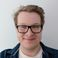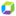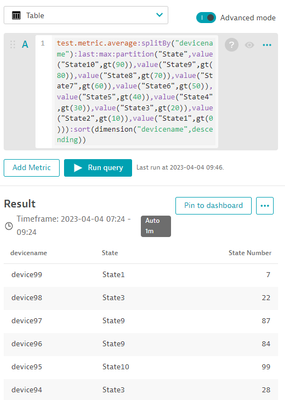cancel
Showing results for
Show  only  | Search instead for
Did you mean:

## Transform metric to stringParticipant

Hi all,

I'm ingesting metrics via api (Metrics API v2).

So, I collect a state (string="OK") and in the script I change for a (integer = 1). The unit type im using is State.
Now in Dynatrace I would like to transform again the metric to string. I have multiple applications with various states so its hard to follow/memorize all the tansformations String<-->Integer.

The challenge that Im facing now is to show the state in a human readable way. I have to create alerts and generate a reasonable dashboard.

1. Is it possible to convert the integer to string inside Dynatrace to generate friendly dashboards? (i have use cases with 10 states 😐)
2. If (1.) its not possible I can use the problem app, but the state of application will be Red/Green.

Thanks all,
RD

2 REPLIES 2Dynatrace Mentor

Hey RD,

In my example below I'm ingesting a metric thats random from 1-99 and has a device name as a dimension. You can then use partitions and a table view to display each dimension (in your case application) as being in a different state based on the last value.The expression I used is as follows:

``test.metric.average:splitBy("devicename"):last:max:partition("State",value("State10",gt(90)),value("State9",gt(80)),value("State8",gt(70)),value("State7",gt(60)),value("State6",gt(50)),value("State5",gt(40)),value("State4",gt(30)),value("State3",gt(20)),value("State2",gt(10)),value("State1",gt(0))):sort(dimension("devicename",descending))``

Hope this helps!Participant

Thank you very much @Fin_Ubels, exactly what I needed 😁